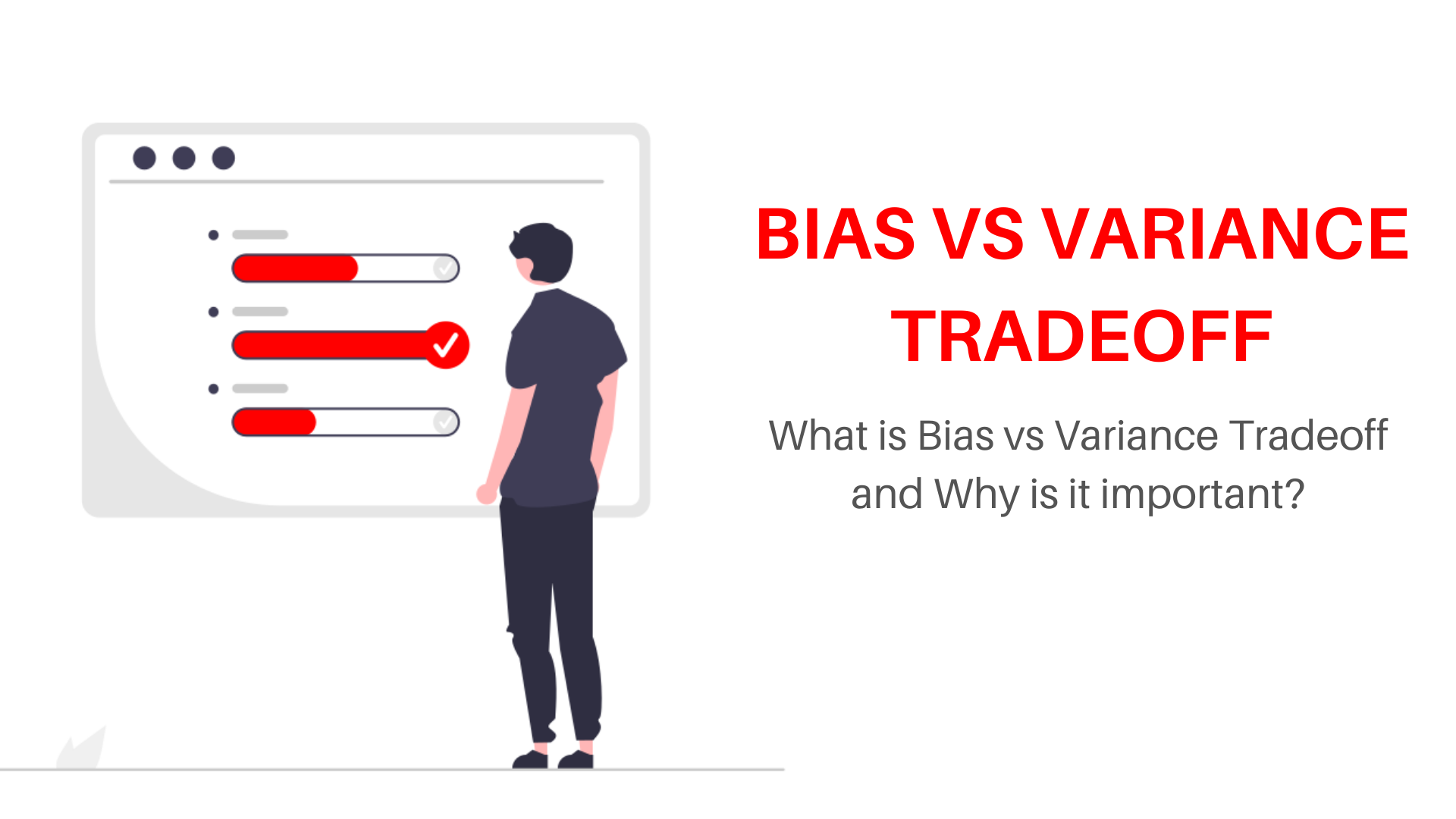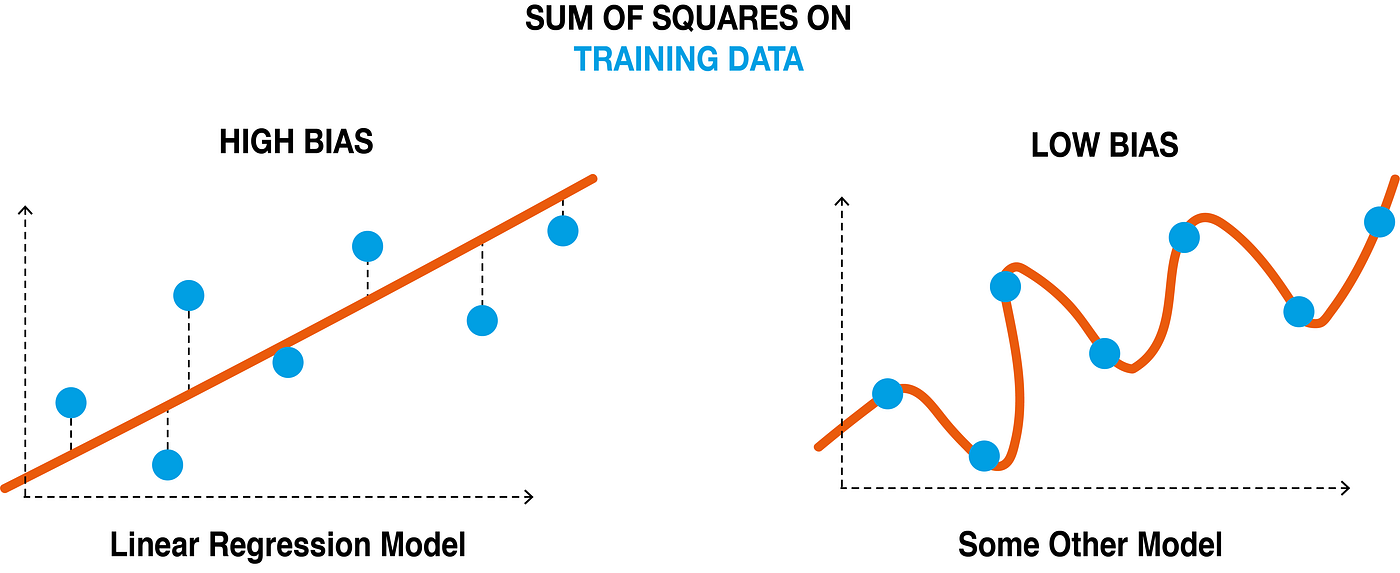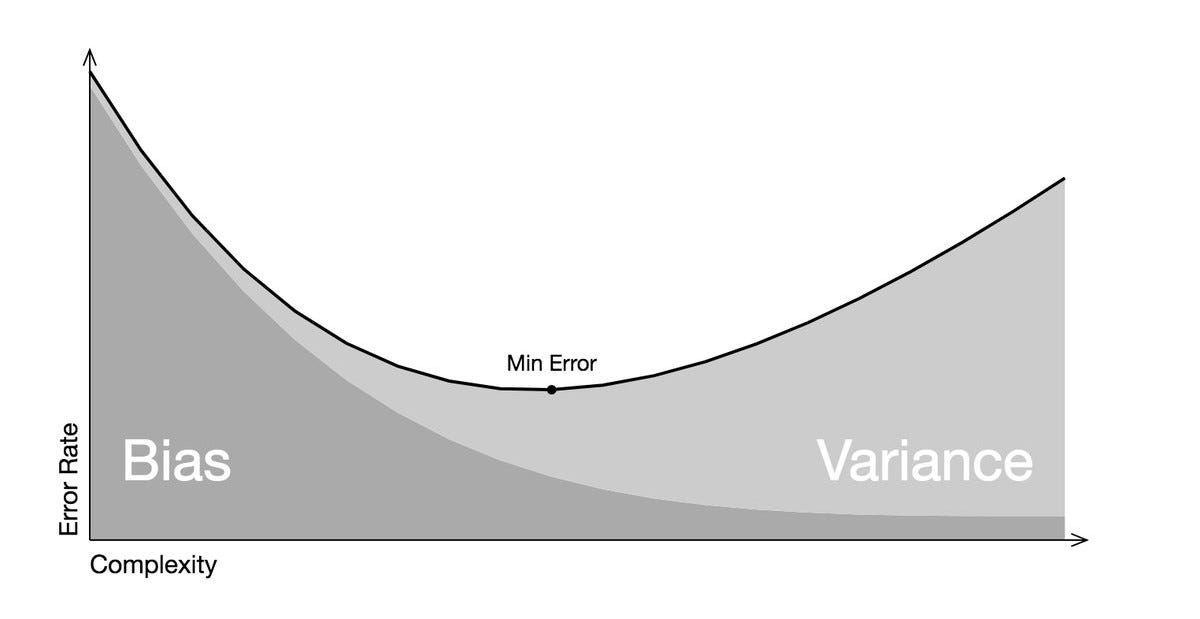# Bias vs Variance Tradeoff: Understanding It The Better Way 2022## Introduction

The bias-variance tradeoff is the central problem in data analytics. When we talk about the bias-variance tradeoff, we are talking about two metrics: bias and variance. The difference between them is that bias represents how much our model misses some points inside the data distribution, while variance describes how much our model misplaces points outside of it. They are called trade-offs because increasing one metric reduces the other and vice versa so we focus on balancing these in such a way that it minimizes the error best it can.

Bias vs Variance tradeoff is an important aspect of building a machine learning model. Sometimes model shows high bias which leads to underfitting and sometimes high variance which leads to overfitting. So there is a need to balance these two and minimize the error. If your model is underperforming then it is likely due to either underfitting or overfitting. Underfitting and Overfitting are two major problems that everyone comes across while building models. These problems arise quite commonly due to several reasons and the main reason behind these two are Bias and Variance.

In the above illustration, we can clearly see high bias and high variance regions which are underfitting and overfitting the model. Also in the adjacent illustration, we can see how the underfitting and overfitting curves deviate from the actual model causing error in the model. In this article, we will discuss this phenomenon and the bias-variance tradeoff.

## Overview

• What is Bias?
• What is Variance?
• How to detect high Bias?
• How to detect high Variance?
• How to prevent high Bias?
• How to prevent high Variance?
• Bias vs Variance Tradeoff
• Conclusion

## What is Bias?

Bias in a machine learning model, also known as algorithmic bias, is nothing but a phenomenon where the model gives more importance to certain features in order to generalize data which leads to error in the model.High vs low bias – source

It is measured by calculating the difference between the average prediction of our model and the correct value that we are trying to predict. More the value of the bias more oversimplification of the model leading to a high error on both training data and testing data.

## What is Variance?

Variance is a measure of dispersion which means it gives us the measure of how far a set of numbers is spread out away from their average value.Notice how variance is increasing – source

The variance of a machine learning model is nothing but the variability of our model prediction for a given data point which tells us the spread of the data. High variance means there is overfitting and doesn’t generalize on the data which it hasn’t seen before. This gives rise to good accuracy on train data but a high error on test data.

## How to detect high Bias?

We can detect the high bias in our model using the learning curves. While training our model we can plot the training accuracy and validation accuracies to check whether our model is generalizing our data or not.

We can clearly see the cases of High bias and Low bias in the above illustration. There is a high training error and also a high validation error in the left graph leading to high bias and on the other side we have a low bias case where the training error is low and also validation error doesn’t converge with the training error. Both are not desirable as we want an ideal case where training accuracy and validation accuracy converge and are almost the same.

So we can say there is high Bias if the training error is high and the validation error is similar in magnitude to the training error.

## How to detect high Variance?

Just like we detected bias in our model, in a similar way we can detect the variance in our model and its effects using the learning curves as shown below

We can clearly see that low variance occurs when there is a high training error and the validation error is also very high. They both don’t seem to diverge instead they seem to be converging. Here initially it seems like an ideal case but this is also not desirable as the error is high and on the other side we see a high variance instance where there is a low training error but a high validation error indicating that our model didn’t generalize the dataset well.

We can conclude that there will be high variance if there is a low training error and high validation error.

Also read: Difference between Knn and K means

## How to prevent high Bias?

High bias in the model leads to underfitting. It can be reduced by using the following methods

• We can add more features by getting more data. This will reduce bias.
• We can also add more polynomial features from the existing features. This will also reduce bias.
• Doing hyperparameter tuning can also help reduce bias as the parameters also affect bias to an extent.
• Regularization also influences bias and decreasing regularization will reduce underfitting.

## How to prevent high Variance?

High Variance in the model leads to overfitting. It can be reduced by using the following methods

• To prevent high variance, we can add more data. This will help reduce variance and can increase generalization.
• Reducing number of features also helps us to decrease the variance and hence the overfitting.
• Increasing regularization will decrease the variance and hence the overfitting. This will increase the accuracy of the model too.

## Bias vs Variance Tradeoff

So far we have seen the concept of bias, variance, and ways to detect them, and also we discussed how to prevent them. However, it is impossible to completely get rid of both bias and variance and have a perfect fit of the training and validation curve. There will always be some error. So how to minimize the error and achieve a good accuracy of the model is an important question. The answer is a tradeoff between bias and variance. Instead of completely getting rid of both bias and variance it is wise to balance them both to minimize the error. It is called a bias vs variance tradeoff.

As we see in the bull’s eye diagram on the right, what we want is to achieve a perfect prediction which is represented in the center of the diagram. But the model doesn’t perform as expected and hence either bias error or variance error occurs which is undesirable. So to balance this error due to bias and error due to variance, we to tradeoff between both and choose the point where these two are optimally low.

In terms of model complexity, as we can see in the above left graph, optimum model complexity is where both bias and variance are low and almost equal to each other and are balanced very well. The error that we see at this point is the lowest error possible and the model performs efficiently at this point. This is how we minimize our model error by doing a bias vs variance tradeoff.

Also read: Confusion matrix

## Conclusion

In this article, we discussed a lot of concepts like bias, variance, the way to detect them, and also we saw what bias vs variance tradeoff is. To decrease overfitting and underfitting of data we do a bias vs variance tradeoff. This bias vs variance tradeoff helps us to achieve optimum model complexity. Hence bias vs variance tradeoff is an important thing while building a machine learning model.##### Aman Kumar

Data Scientist with 3+ years of experience in building data-intensive applications in diverse industries. Proficient in predictive modeling, computer vision, natural language processing, data visualization etc. Aside from being a data scientist, I am also a blogger and photographer.